# Chapter 1.ppt

May. 28, 2023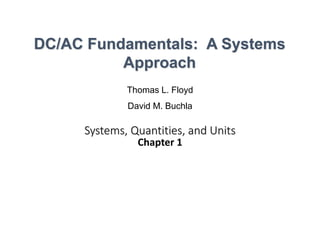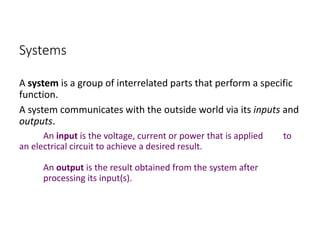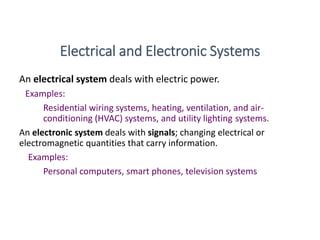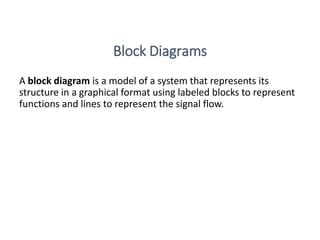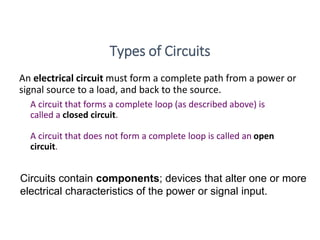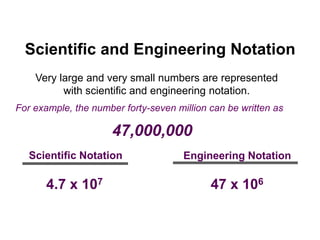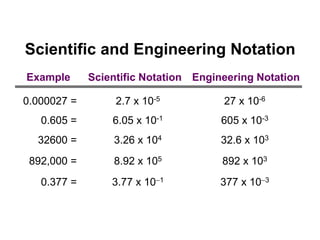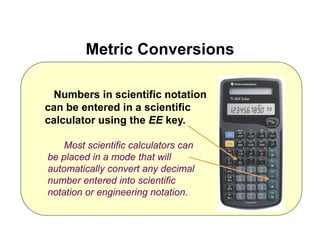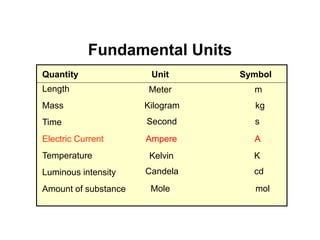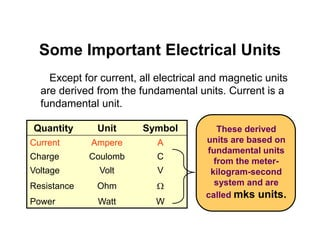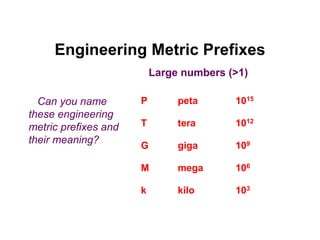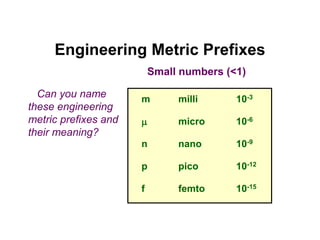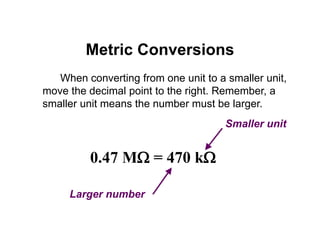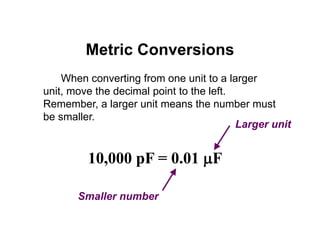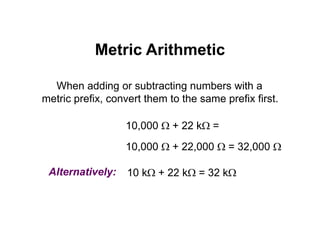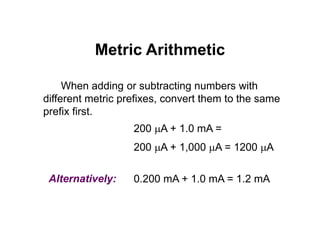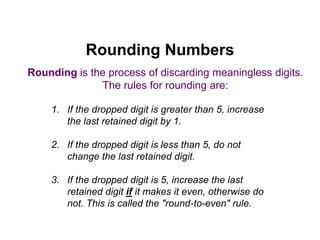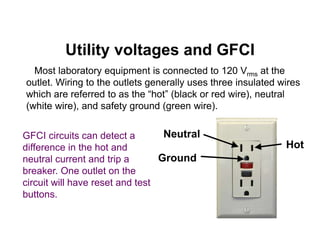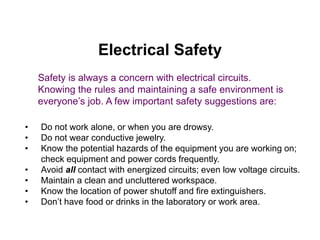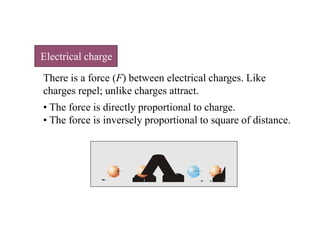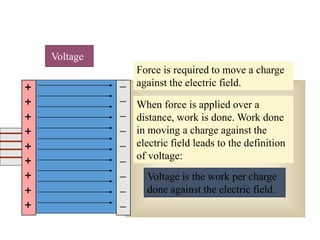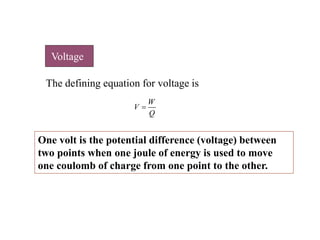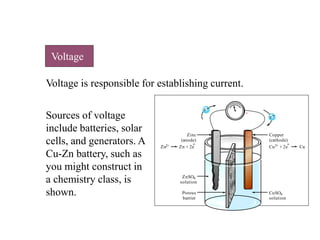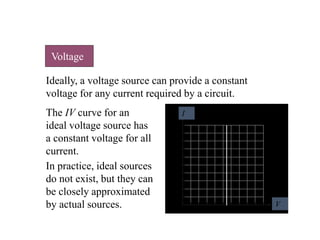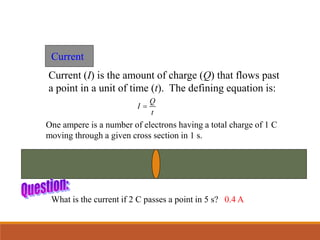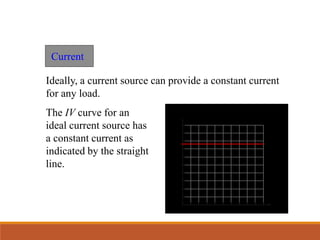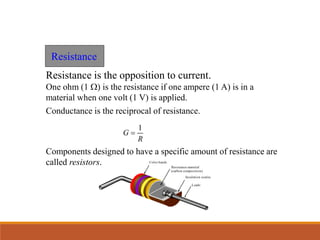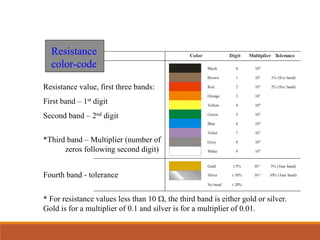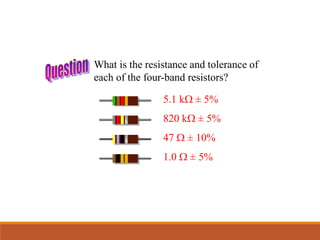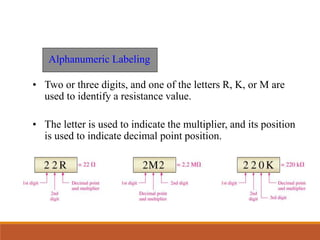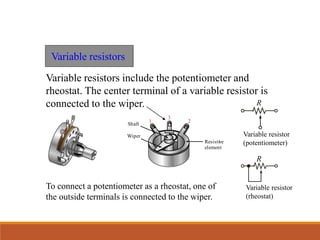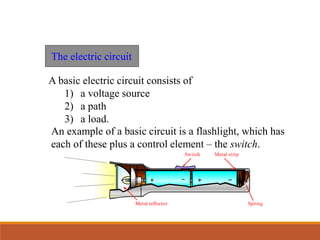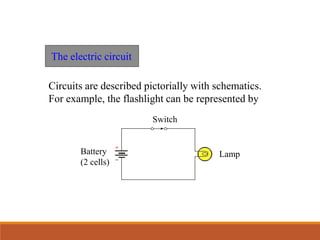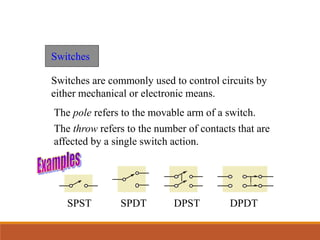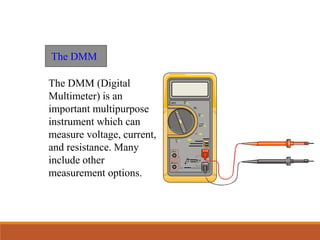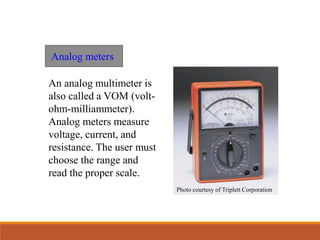1 of 36

### Chapter 1.ppt

• 1. Systems, Quantities, and Units Chapter 1 Thomas L. Floyd David M. Buchla DC/AC Fundamentals: A Systems Approach
• 2. Systems A system is a group of interrelated parts that perform a specific function. A system communicates with the outside world via its inputs and outputs. An input is the voltage, current or power that is applied to an electrical circuit to achieve a desired result. An output is the result obtained from the system after processing its input(s).
• 3. An electrical system deals with electric power. Examples: Residential wiring systems, heating, ventilation, and air- conditioning (HVAC) systems, and utility lighting systems. An electronic system deals with signals; changing electrical or electromagnetic quantities that carry information. Examples: Personal computers, smart phones, television systems Electrical and Electronic Systems
• 4. A block diagram is a model of a system that represents its structure in a graphical format using labeled blocks to represent functions and lines to represent the signal flow. Block Diagrams
• 5. An electrical circuit must form a complete path from a power or signal source to a load, and back to the source. A circuit that forms a complete loop (as described above) is called a closed circuit. A circuit that does not form a complete loop is called an open circuit. Types of Circuits Circuits contain components; devices that alter one or more electrical characteristics of the power or signal input.
• 6. Very large and very small numbers are represented with scientific and engineering notation. Scientific and Engineering Notation 4.7 x 107 Scientific Notation 47 x 106 Engineering Notation For example, the number forty-seven million can be written as 47,000,000
• 7. 2.7 x 10-5 Scientific and Engineering Notation Scientific Notation Engineering Notation Example 0.000027 = 27 x 10-6 0.605 = 6.05 x 10-1 605 x 10-3 32600 = 3.26 x 104 32.6 x 103 892,000 = 8.92 x 105 892 x 103 0.377 = 3.77 x 101 377 x 103
• 8. Numbers in scientific notation can be entered in a scientific calculator using the EE key. Metric Conversions Most scientific calculators can be placed in a mode that will automatically convert any decimal number entered into scientific notation or engineering notation.
• 9. Fundamental Units Quantity Unit Symbol Length Mass Time Electric Current Temperature Luminous intensity Amount of substance Meter m Kilogram kg Second s Ampere A Kelvin K Candela cd Mole mol
• 10. Except for current, all electrical and magnetic units are derived from the fundamental units. Current is a fundamental unit. These derived units are based on fundamental units from the meter- kilogram-second system and are called mks units. Some Important Electrical Units Quantity Unit Symbol Current Ampere A Charge Coulomb C Voltage Volt V Resistance Ohm W Power Watt W
• 11. peta tera giga mega kilo 1015 1012 109 106 103 P T G M k Can you name these engineering metric prefixes and their meaning? Engineering Metric Prefixes Large numbers (>1)
• 12. milli micro nano pico femto 10-3 10-6 10-9 10-12 10-15 m m n p f Can you name these engineering metric prefixes and their meaning? Engineering Metric Prefixes Small numbers (<1)
• 13. When converting from one unit to a smaller unit, move the decimal point to the right. Remember, a smaller unit means the number must be larger. 0.47 MW = 470 kW Metric Conversions Smaller unit Larger number
• 14. When converting from one unit to a larger unit, move the decimal point to the left. Remember, a larger unit means the number must be smaller. 10,000 pF = 0.01 mF Metric Conversions Larger unit Smaller number
• 15. When adding or subtracting numbers with a metric prefix, convert them to the same prefix first. 10,000 W + 22 kW = 10,000 W + 22,000 W = 32,000 W Alternatively: 10 kW + 22 kW = 32 kW Metric Arithmetic
• 16. When adding or subtracting numbers with different metric prefixes, convert them to the same prefix first. 200 mA + 1.0 mA = 200 mA + 1,000 mA = 1200 mA Alternatively: 0.200 mA + 1.0 mA = 1.2 mA Metric Arithmetic
• 17. 1. If the dropped digit is greater than 5, increase the last retained digit by 1. 2. If the dropped digit is less than 5, do not change the last retained digit. 3. If the dropped digit is 5, increase the last retained digit if it makes it even, otherwise do not. This is called the "round-to-even" rule. Rounding is the process of discarding meaningless digits. The rules for rounding are: Rounding Numbers
• 18. Most laboratory equipment is connected to 120 Vrms at the outlet. Wiring to the outlets generally uses three insulated wires which are referred to as the “hot” (black or red wire), neutral (white wire), and safety ground (green wire). Neutral Hot Ground GFCI circuits can detect a difference in the hot and neutral current and trip a breaker. One outlet on the circuit will have reset and test buttons. Utility voltages and GFCI
• 19. • Do not work alone, or when you are drowsy. • Do not wear conductive jewelry. • Know the potential hazards of the equipment you are working on; check equipment and power cords frequently. • Avoid all contact with energized circuits; even low voltage circuits. • Maintain a clean and uncluttered workspace. • Know the location of power shutoff and fire extinguishers. • Don’t have food or drinks in the laboratory or work area. Safety is always a concern with electrical circuits. Knowing the rules and maintaining a safe environment is everyone’s job. A few important safety suggestions are: Electrical Safety
• 20. There is a force (F) between electrical charges. Like charges repel; unlike charges attract. • The force is directly proportional to charge. • The force is inversely proportional to square of distance. + + + _ Electrical charge
• 21. e- Force is required to move a charge against the electric field. Voltage is the work per charge done against the electric field. Voltage + + + + + + + + +          When force is applied over a distance, work is done. Work done in moving a charge against the electric field leads to the definition of voltage:
• 22. W V Q  One volt is the potential difference (voltage) between two points when one joule of energy is used to move one coulomb of charge from one point to the other. Voltage The defining equation for voltage is
• 23. Voltage is responsible for establishing current. Sources of voltage include batteries, solar cells, and generators. A Cu-Zn battery, such as you might construct in a chemistry class, is shown. Zinc (anode) Zn + 2e Zn2+ ZnSO4 solution Porous barrier Copper (cathode) Cu2+ + 2e CuSO4 solution Cu + e e - - - - Voltage
• 24. Ideally, a voltage source can provide a constant voltage for any current required by a circuit. In practice, ideal sources do not exist, but they can be closely approximated by actual sources. Voltage I V The IV curve for an ideal voltage source has a constant voltage for all current.
• 25. Current (I) is the amount of charge (Q) that flows past a point in a unit of time (t). The defining equation is: Q I t  One ampere is a number of electrons having a total charge of 1 C moving through a given cross section in 1 s. 0.4 A What is the current if 2 C passes a point in 5 s? Current
• 26. Ideally, a current source can provide a constant current for any load. Current I V The IV curve for an ideal current source has a constant current as indicated by the straight line.
• 27. Resistance is the opposition to current. One ohm (1 W) is the resistance if one ampere (1 A) is in a material when one volt (1 V) is applied. Conductance is the reciprocal of resistance. 1 G R  Components designed to have a specific amount of resistance are called resistors. Color bands Resistance material (carbon composition) Insulation coating Leads Resistance
• 28. Color Black Brown Red Orange Yellow Green Blue Violet Gray White Gold Silver No band 0 1 2 3 4 5 6 7 8 9 ±5% ± 10% Digit ± 20% 100 101 102 103 104 105 106 107 108 109 10-1 10-2 Multiplier 1% (five band) 5% (four band) T olerance 2% (five band) 10% (four band) Resistance value, first three bands: First band – 1st digit Second band – 2nd digit *Third band – Multiplier (number of zeros following second digit) Fourth band - tolerance * For resistance values less than 10 W, the third band is either gold or silver. Gold is for a multiplier of 0.1 and silver is for a multiplier of 0.01. Resistance color-code
• 29. What is the resistance and tolerance of each of the four-band resistors? 5.1 kW ± 5% 820 kW ± 5% 47 W ± 10% 1.0 W ± 5%
• 30. • Two or three digits, and one of the letters R, K, or M are used to identify a resistance value. • The letter is used to indicate the multiplier, and its position is used to indicate decimal point position. Alphanumeric Labeling
• 31. Variable resistors include the potentiometer and rheostat. The center terminal of a variable resistor is connected to the wiper. 1 3 2 Resistive element Wiper Shaft Variable resistors R Variable resistor (potentiometer) R Variable resistor (rheostat) To connect a potentiometer as a rheostat, one of the outside terminals is connected to the wiper.
• 32. A basic electric circuit consists of 1) a voltage source 2) a path 3) a load. Switch Metal strip Metal reflector Spring The electric circuit An example of a basic circuit is a flashlight, which has each of these plus a control element – the switch.
• 33. The electric circuit Circuits are described pictorially with schematics. For example, the flashlight can be represented by Battery (2 cells) Switch Lamp
• 34. Switches The pole refers to the movable arm of a switch. Switches are commonly used to control circuits by either mechanical or electronic means. The throw refers to the number of contacts that are affected by a single switch action. SPST SPDT DPST DPDT
• 35. The DMM (Digital Multimeter) is an important multipurpose instrument which can measure voltage, current, and resistance. Many include other measurement options. The DMM V Hz 10 A 40 mA OFF mV A V H H V H COM VW
• 36. An analog multimeter is also called a VOM (volt- ohm-milliammeter). Analog meters measure voltage, current, and resistance. The user must choose the range and read the proper scale. Analog meters Photo courtesy of Triplett Corporation